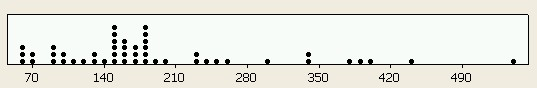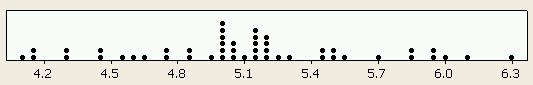# Graph and Calculator for Confidence Intervals for Task Times

Jeff Sauro, PhD

This calculator takes raw task times, transforms them using the Natural Logarithm and computes a confidence interval. The values are displayed in the dot-plots graph below. You can also download an excel version of this calculator.

### Results

95% Confidence Interval: ( 35.5, 59.3 ) Geometric Mean: 45.87
Arithmetic Mean: 50.09 Median: 39
Arithmetic StDev: 29.05 Observations: 11Reporting Results

Use the low and high values in the Results section as the confidence intervals for the task times. These values correspond to the green-dashed lines in the the graphs. They are the boundaries of the confidence interval. For example, if you entered the raw task times: 55, 65, 75, 62, 45, 135 (in any order) with a 95% confidence level, you would report the following:
• Average Time: 68 seconds, 95% CI (46,101)
The arithmetic mean is provided as a point of reference. You'll notice how the geometric mean is lower than the arithmetic mean, this is a symptom of a positively skewed distribution: the more your data is positively skewed the bigger the difference between the two means. If your data is normally distributed, the two values would be almost identical.

One consequence of using the transformed values to derive the confidence interval is that the intervals are not symmetric around the mean. You'll notice in the example above that the margin of error, is 68 + 33 seconds and 68 - 22 seconds. This asymmetry is caused by the nonlinear log transformation.

Raw Values Format
Raw values should be the task times in seconds format (e.g. two minutes 15 seconds = 135). Minutes and seconds separated by a colon will not work (e.g. 2:30 will return an error). You can enter up to 20 values in any order.

Geometric Mean
The geometric mean should be used instead of the arithmetic mean since values from time data are almost always positively skewed. The geometric mean is the exponentiated value of the arithmetic mean of the natural logged values. In English, if you take the raw task times, convert them into logged values, take the average of these values then convert this log average back into raw form(called exponentiate), you have the Geometric Mean. Exponentiate is like taking the anti-log of a logged value. You anti-log a value when the logarithm is base 10, you exponentiate a value when the logarithm is natural or base e (approx 2.71828). The Geometric Standard deviation is a misleading figure, so I don't recommend reporting that with the Geometric Mean, instead, report the confidence intervals.

Why do I need to Transform?

It's nothing personal, but your task time data probably isn't normally distributed. Don't worry, it's normal for task time data not-to be normal (ok, enough with the double use of "normal"). Task time data, like most time data is positively skewed. This skewness comes from two major elements:

1. There is a natural lower boundary in times (it's physically impossible to complete a task faster than some minimum time).
2. Some users will take an exceptionally long amount of time to complete the task.
You can see the skewness by plotting your data. Figure 1 below shows raw task times from a large usability study with 49 users. Notice how the times have a lower boundary at around 70 seconds and the long times above 400 seconds. Figure 2 shows what happens with the log-transformation. This transformation takes the longer task times and pulls them in. Also notice how the distribution looks more like a normal "bell-curve."

Most confidence interval formulas need the data to be approximately normally distributed for their endpoints to be accurate. The log-transformation has been found to be one of the best transformations for time data (e.g. Howell p.346) since the means and standard deviations are correlated.

Figure 1: Raw TimeFigure 2: Log-Transformed TimesShould I report the Geometric Mean or Median?

The short answer is report the Geometric Mean. The more complicated and technical answer is : With small samples there is evidence that the Median is less accurate than the mean, since the sample median is not considered an unbiased estimate of the population median (Cordes 1993), (Eisenhart et al 1948). Now, when data are transformed as they are in the above calculations, an interesting situation occurs. The log of the skewed values will create a more symmetric distribution as Figures 1 and 2 show. If the distribution becomes symmetric the mean and the median are identical. Therefore, when we take the mean of the logged values this should roughly equal the raw median. This isn't always the case due to odd or even values affecting the median. Since the Geomtric Mean will rarely be identical to the Median, it's better to use the Geometric Mean, for the same reason the Arithmetic mean is better than the Median with small samples. There is an excellent discussion of this issue here: Summary Statistics:Location & Spread

References

Howell, David C. (2002) "Statistical Methods for Psychology" Fifth Edition. Thomas Learning.

Cordes, Richard (1993) "The Effects of Running Fewer Subjects on Time-on-Task Measures" Internation Journal of Human Computer Interaction 5(4) 393-403.

Eisenhart, C, Deming L, & Martin CS (1948) "On the Aritmetic Mean and Median in Small Samples from the Normal and Certain Non-Normal Populations." Annals of Mathematical Statistics p599

0
0